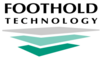NYC CCoC Evaluation Tool Data Fiscal | NYC CCoC Evaluation Tool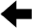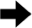Below is a detailed list of the questions located on the NYC CCoC Evaluation Tool report and the data they contain.  To jump directly to the information for a specific question, press <Ctrl+F> to access your browser's search field and enter the name of the question you'd like to view.

Q1. Unit Utilization Rate

Description:

"Average daily unit utilization rate during most recently completed HUD contract (Projects for singles will use Projected # of Beds, and projects for families will use Projected # of Units)."

Applicable project types:

Permanent Supportive Housing and Transitional Housing

HUD benchmark:

85%

How it's calculated:

Behind the scenes, this Tool runs the Housing Utilization Report in AWARDS to get the actual unit utilization rate. The Unit Utilization Rate is based on the number of bed nights for primary clients, divided by the Projected # of Units entered in Agency Program Information. (NYCHMIS does not use the Residence Units feature.)

Point breakdowns:

Less than 80.00% = 0 points
80.00% to 81.24% = 1 point
81.25% to 82.49% = 1.5 points
82.50% to 83.74% = 2 points
83.75% to 84.49% = 2.5 points
84.50% to 86.24% = 3 points
86.25% to 87.49% = 3.5 points
87.50% to 88.74% = 4 points
88.75% to 89.49% = 4.5 points
89.50% to 90.49% = 5 points
90.50% to 91.49% = 6 points
91.50% to 92.49% = 7 points
92.50% to 93.49% = 8 points
93.50% to 94.49% = 9 points
94.50% to 95.49% = 10 points
95.50% to 96.49% = 11 points
96.50% to 97.49% = 12 points
97.50% to 98.49% = 13 points
98.50% to 99.49% = 14 points
99.50% or higher = 15 points

Q2. Serving chronically homeless

Description:

"% of new HoH who entered the program that are chronically homeless at placement into the program. Source: Intake/Admission data"

Applicable project types:

Permanent Supportive Housing

HUD benchmark:

100%

How it's calculated:

This question looks at the "Chronically Homeless (Admission)" field on the HMIS ReportBuilder for all clients with admission dates that fall within the reporting period.  If 100% of clients are Chronically Homeless, then full points are given.  If no clients were admitted during the reporting period, then full points are given.

Point breakdowns:

Less than 100% = 0 points
100% = 5 points

Q3. Participants entering program are literally homeless

Description:

"% of HoH in the program that are literally homeless. Source: Intake/Admission Living Situation Fields."

Applicable project types:

Permanent Supportive Housing, Transitional Housing

HUD benchmark:

100%

How it's calculated:

This question checks Intake/Admission for clients who have admission dates of 1/4/2012 or after.  There it looks to see if there is either a "Prior Living Situation: Type of Residence" answer of "Emergency shelter, including hotel or motel paid for with emergency shelter voucher," "Transitional housing for homeless persons," "Place not meant for habitation," or "Safe Haven," or a "yes" to "On the night before, did you stay on the street" answer. If one of those was true, the client is considered "homeless at admission," and the ratio of these clients to total clients is a "project's performance."

Point breakdowns:

Less than 100% = 0 points
100% = 5 points

Q4. Average length of stay

Description:

"Average length of stay for participants served during recently completed FFY. Source: APR Q22."

Applicable project types:

Permanent Supportive Housing, Transitional Housing

HUD benchmark:

Less than or equal to 24 months for TH; Greater than or equal to 12 months for PSH.

How it's calculated:

This question first takes the sum of (APR Q22b "Average Length of Stay for Leavers" multiplied by APR Q5 "Number of Leavers") and (APR Q22b "Average Length of Stay for Stayers" multiplied by APR Q5 "Number of Stayers").  This number is then divided by APR Q5 "Total number of Persons Served" to get the Average Length of Stay in Days.  This number is then divided by 30.41 to get the Average Length of Stay in Months.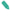NOTE: Average length of stay is rounded to the nearest whole number.

Point breakdowns for Transitional Housing:

More than 24 months = 0 Points
24 months or less = 1 point
23 months or less = 1.5 points
22 months or less = 2 points
21 months or less = 2.5 points
20 months or less = 3 points
19 months or less = 3.5 points
18 months or less = 4 points
17 months or less = 4.5 points
16 months or less = 5 points
15 months or less = 5.5 points
14 months or less = 6 points
13 months or less = 6.5 points
12 months or less = 7 points

Point breakdowns for Permanent Supportive Housing:

Less than 12 months = 0 points
12 months or more = 1 point
13 months or more = 1.5 points
14 months or more = 2 points
15 months or more = 2.5 points
16 months or more = 3 points
17 months or more = 3.5 points
18 months or more = 4 points
19 months or more = 4.5 points
20 months or more = 5 points
21 months or more = 5.5 points
22 months or more = 6 points
23 months or more = 6.5 points
24 months or more = 7 points

Q5. Gained or Increase earned income - adult stayers and adult exiters

Description:

"% of adults that increased earned income at latest status or exit. Source: APR Q19"

Applicable project types:

Permanent Supportive Housing, Transitional Housing

HUD benchmark:

54%

How it's calculated:

This question uses APR Q19a1 and Q19a2.  In the row labeled "Number of Adults with Earned Income (i.e., Employment Income)," start by adding the sum of the columns "Retained Income Category and Increased \$ at [Annual Assessment/Exit]" and "Did Not Have the Income Category at Start and Gained the Income Category at [Annual Assessment/Exit]."  This gives you the total adults who increased their earned income.  This number is then divided by the difference of APR Q5 "Number of Adults" minus APR Q18 "Number of adult stayers not yet required to have an annual assessment."

Point breakdowns:

Less than 50% = 0 points
50% = 1 point
51% = 1.5 points
52% = 2 points
53% = 2.5 points
54% = 3 points
55% = 3.5 points
56% = 4 points
57% = 4.5 points
58% or 59% = 5 points
60% = 6 points
61% = 6.5 points
62% = 7 points
63% = 7.5 points
64% = 8 points
65% = 8.5 points
66% = 9 points
67% = 9.5 points
68% = 10 points
69% = 10.5 points
70% = 11 points
71% = 11.5 points
72% = 12 points
73% = 12.5 points
74% = 13 points
75% = 13.5 points
76% = 14 points
77% = 14.5 points
78% = 15 points
79% = 15.5 points
80% = 16 points
81% = 16.5 points
82% = 17 points
83% = 17.5 points
84% or higher = 18 points

Q6. Non-cash benefits- adult stayer and all exiters

Description:

"% of persons with 1 or more sources of non cash benefits at latest status or exit. Source: APR Q20b"

Applicable project types:

Permanent Supportive Housing, Transitional Housing

HUD benchmark:

75%

How it's calculated:

This question uses APR Q20b "Number of Non-Cash Benefit Sources."  In the row labeled "1+ Source(s)," start by adding the sum of the columns "Benefit at Latest Annual Assessment for Stayers" and "Benefit at Exit for Leavers."  This gives you the total adults with Non-Cash Benefits at their latest stage.  This number is then divided by the difference of APR Q5 "Number of Adults," minus APR Q18 "Number of adult stayers not yet required to have an annual assessment."

Point breakdowns:

Less than 70% = 0 Points
70.00% to 72.49% = 1 Point
72.50% to 74.49% = 1.5 Points
74.50% to 77.49% = 2 Points
77.50% to 79.49% = 2.5 Points
79.50% to 82.49% = 3 Points
82.50% to 84.49% = 3.5 Points
84.50% to 86.49% = 4 Points
86.50% to 87.49% = 4.5 Points
87.50% to 89.49% = 5 Points
89.50% to 90.49% = 5.5 Points
90.50% to 92.49% = 6 Points
92.50% to 93.49% = 6.5 Points
93.50% to 95.49% = 7 Points
95.50% to 96.49% = 7.5 Points
96.50% to 98.49% = 8 Points
98.50% to 99.49% = 8.5 Points
99.50% to 100% = 9 Points

Q7. Health Insurance

Description:

"% of persons with health insurance. Source: APR Q21"

Applicable project types:

Permanent Supportive Housing, Transitional Housing

HUD benchmark:

75%

How it's calculated:

This question uses APR Q21 "Health Insurance."  In the rows labeled "1 Source of Health Insurance" and "More than 1 Source of Health Insurance," add the sums of the columns "at Latest Annual Assessment for Stayers" and "at Exit for Leavers."  The sum of these four cells gives you the total persons with Health Insurance at their latest stage.  This number is then divided by the difference of APR Q5 "Total Number of Persons Served," minus APR Q21 "Number of stayers not yet required to have an annual assessment."

Point breakdowns:

Less than 75% = 0 Points
75% to 77.49% = 1 Point
77.50% to 79.49% = 1.5 Points
79.50% to 82.49% = 2 Points
82.50% to 84.49% = 2.5 Points
84.50% to 87.49% = 3 Points
87.50% to 89.49% = 3.5 Points
89.50% to 92.49% = 4 Points
92.50% to 94.49% = 4.5 Points
94.50% to 97.49% = 5 Points
97.50% to 99.49% = 5.5 Points
99.50% to 100% = 6 Points

Q8. Exiting to Permanent Housing (TH) / Maintain Permanent Housing or exit to Permanent Housing (PSH)

When run for a Transitional Housing (TH) program

Description:

"% of leavers exiting to permanent housing.  Source: APR Q23a+Q23b"

Applicable project types:

Transitional Housing

HUD benchmark:

75%

How it's calculated:

This question uses APR Q23a "Exit Destination - More than 90 Days" and Q23b "Exit Destination - 90 Days or Less."  Add the sum of the Q23a "Total persons exiting to positive housing destinations" from Q23a and Q23b.  This number is then divided by (Q23a "Total" - Q23a "Total persons whose destinations excluded them from the calculation" + Q23b "Total" - Q23b "Total persons whose destinations excluded them from the calculation").  The resulting percentage gives you the total number of successful exits divided by the total number of leavers excluding those who exited to destinations that are excluded from the calculation.

Point breakdowns:

Less than 70% = 0 Points
70.00% to 71.24% = 1 Points
71.25% to 72.49% = 1.5 Points
72.50% to 73.74% = 2 Points
73.75% to 74.49% = 2.5 Points
74.50% to 76.24% = 3 Points
76.25% to 77.49% = 3.5 Points
77.50% to 78.74% = 4 Points
78.75% to 79.49% = 4.5 Points
79.50% to 81.24% = 5 Points
81.25% to 82.49% = 5.5 Points
82.50% to 83.74% = 6 Points
83.75% to 84.49% = 6.5 Points
84.50% to 86.24% = 7 Points
86.25% to 87.49% = 7.5 Points
87.50% to 88.74% = 8 Points
88.75% to 89.49% = 8.5 Points
89.50% to 90.49% = 9 Points
90.50% to 91.49% = 9.5 Points
91.50% to 92.49% = 10 Points
92.50% to 93.49% = 10.5 Points
93.50% to 94.49% = 11 Points
94.50% to 95.49% = 11.5 Points
95.50% to 96.49% = 12 Points
96.50% to 97.49% = 12.5 Points
97.50% to 98.49% = 13 Points
98.50% to 99.49% = 13.5 Points
99.50% to 100% = 14 Points

When run for a Permanent Housing (PSH) program

Description:

"% of participants who remain in PSH or exit to permanent housing. Source: APR Q5 and Q23a+Q23b"

Applicable project types:

Permanent Supportive Housing

HUD benchmark:

90%

How it's calculated:

This question uses APR Q23a "Exit Destination - More than 90 Days" and Q23b "Exit Destination - 90 Days or Less."  Add the sum of "Total persons exiting to positive housing destinations" from Q23a and Q23b along with Q5 "Number of Stayers."  This number is then divided by (Q5 "Total Number of Persons Served" - Q23a "Total persons whose destinations excluded them from the calculation" - Q23b "Total persons whose destinations excluded them from the calculation").  The resulting percentage gives you the total number of stayers + successful exits, divided by the total number of people excluding those who exited to destinations that are excluded from the calculation.  The resulting percentage is rounded to the nearest whole number.

Point breakdowns:

Less than 85% = 0 Points
85.00% to 85.49% = 1 Point
85.50% to 86.49% = 1.5 Points
86.50% to 87.49% = 2 Points
87.50% to 88.49% = 2.5 Points
88.50% to 89.49% = 3 Points
89.50% to 90.49% = 3.5 Points
90.50% to 91.49% = 4 Points
91.50% to 92.49% = 4.5 Points
92.50% to 93.49% = 5 Points
93.50% to 94.49% = 5.5 Points
94.50% to 95.49% = 6 Points
95.50% to 96.49% = 6.5 Points
96 .50% to 97.49% = 7 Points
97.50% to 98.49% = 8 Points
98.50% to 99.49% = 9 Points
99.50% to 100% = 10 Points

Q9. Exit to Permanent Housing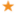IMPORTANT! This question is only displayed when the report is run for a Permanent Supportive Housing program.  When run for a Transitional Housing program, the report skips from Q8 to Q10a.

Description:

"% of leavers who exit to permanent housing. Source: APR Q23a+Q23b"

Applicable project types:

Permanent Supportive Housing

HUD benchmark:

N/A

How it's calculated:

This question uses APR Q23a "Exit Destination - More than 90 Days" and Q23b "Exit Destination - 90 Days or Less."  Add the sum of the Q23a "Total persons exiting to positive housing destinations" from Q23a and Q23b.  This number is then divided by (Q23a "Total" - Q23a "Total persons whose destinations excluded them from the calculation" + Q23b "Total" - Q23b "Total persons whose destinations excluded them from the calculation").  The resulting percentage gives you the total number of successful exits, divided by the total number of leavers excluding those who exited to destinations that are excluded from the calculation.  If no clients are discharged then full points are given.

Point breakdowns:

Less than 25% = 0 Points
25.00% to 37.49% = 1 Point
37.50% to 49.49% = 1.5 Points
49.50% to 62.49% = 2 Points
62.50% to 74.49% = 2.5 Points
74.50% to 87.49% = 3 Points
87.50% to 99.49% = 3.5 Points
99.50% to 100% = 4 Points

Q10a. Data Quality

Description:

"One point if the overall missing or invalid data is less than or equal to 10%. Source: APR Q6a"

Applicable project types:

Permanent Supportive Housing and Transitional Housing

HUD benchmark:

N/A

How it's calculated:

This question reports the "Overall Score" from Q6a "Data Quality - Personally Identifiable Information."

Point breakdowns:

Higher than 10% = 0 points
10% or less = 1 point

Q10b. Data Quality

Description:

"One point if the overall missing or invalid data is less than or equal to 10%. Source: APR Q6b"

Applicable project types:

Permanent Supportive Housing and Transitional Housing

HUD benchmark:

N/A

How it's calculated:

This question reports the average of all 5 data elements listed in Q6b "Data Quality - Universal Data Elements."

Point breakdowns:

Higher than 10% = 0 points
10% or less = 1 point

Q10c. Data Quality

Description:

"One point if the overall missing or invalid data is less than or equal to 10%. Source: APR Q6c"

Applicable project types:

Permanent Supportive Housing and Transitional Housing

HUD benchmark:

N/A

How it's calculated:

This question reports the average of all 4 data elements listed in Q6c "Data Quality - Income and Housing Data Quality."

Point breakdowns:

Higher than 10% = 0 points
10% or less = 1 point

Q10d. Data Quality

Description:

"One point if the overall missing or invalid data is less than or equal to 10%. Source: APR Q6d"

Applicable project types:

Permanent Supportive Housing and Transitional Housing

HUD benchmark:

N/A

How it's calculated:

This question reports the "% of Records Unable to Calculate" from Q6d "Data Quality - Chronic Homelessness" from the "Total" row.

Point breakdowns:

Higher than 10% = 0 points
10% or less = 1 point

Q11. Has the program performed 12 monthly uploads during the reporting year

Description:

"Verification of a minimum of 12 monthly uploads to HMIS. Minus 2.5 points if one upload is late or missing. Minus 5 points if more than one upload is late or missing."

Applicable project types:

Permanent Supportive Housing and Transitional Housing

HUD benchmark:

N/A

How it's calculated:

This question looks at the CSV Upload Report in the Fiscal Program menu to count the number of monthly uploads that occurred during the operating year.  The date of the first upload from each month is evaluated to verify that it happened within the first 10 business days of the month excluding weekends and federal holidays.

Point breakdowns:

12 Monthly Uploads = 0 points
11 Monthly Uploads = -2.5 points
10 or Fewer Monthly Uploads = -5 points

https://demodb.footholdtechnology.com/help/?12240